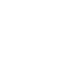# Apr 28 SAT Question of the Day & ACT QotD

If you are reading this in an email you received from me, do not click the link to sat.collegeboard.org below. Use the link to my website that is farther down on the email. If you are seeing this in my blog, do the SAT Question of the Day by clicking on this link:

http://sat.collegeboard.org/practice/sat-question-of-the-day?src=R&questionId=20130428 (This link takes you to today’s question. If you use my archive, you will see the question related to my SAT explanation for that date.)

The answer is B.  Anytime you have to divide by 0, y is “not defined.”  That is because you cannot divide by 0.  So, just figure out how the denominator could be 0.  You know that 0 times anything is 0.  That means that if either binomial in the denominator is 0, then the whole denominator becomes 0 when you multiply the binomials times one another.  If x= -3, then the left-hand binomial is 0 and y is undefined, that’s Answer B.  Another possibility is if x=4, then the right hand binomial in the denominator is 0 and then the whole denominator when you multiply is 0.  So, 4 could be a right answer but it isn’t an option!

This question’s math isn’t hard; it was just a matter of knowing the definition of “undefined.”  You also needed to recognize a math concept that shows up a lot on the SAT and ACT: multiplication by 0.  (0 times anything is 0.)  Watch for it and you’re score will improve.

Let’s see what the ACT folks have for us this morning.

http://www.act.org/qotd/ (The ACT staff does not put a date on their questions so if you click on an archived blog, you’ll get today’s question and the old explanation. Sorry. The SAT staff has dated their questions; so, the archive is helpful. The ACT folks simply don’t do that.)

The answer is J.  This is not so much of a geometry question as it is a ratio question.  In fact, it is a “hybrid” between the two.  You have to know one of the Wizard’s Keys which is: “The key to all ratio questions is the sum of the parts.”  So, begin by adding 2+3+5 equals 10 parts.  The smallest angle is 2/10 or 1/5 of the whole.  The whole is 180 degrees. (That’s where the geometry comes in.)  2/10 or 1/5 of 180 degrees is 36.  All done.

Enjoy your Sunday.  I’m looking forward to both of my groups of students today and tutoring some individual students.  It is going to be a great day.  I hope yours is as rewarding.

Wizard